Get ICSE Solutions for Class 10 Mathematics Chapter 3 Banking for ICSE Board Examinations on ICSESolutions.com. We provide step by step Solutions for ICSE Mathematics Class 10 Solutions Pdf. You can download the Class 10 Maths ICSE Textbook Solutions with Free PDF download option.

## Banking Class 10 Maths ICSE Solutions

### Formulae

Calculating interest on a savings bank account:

1. Interest for the month is calculated on the minimum balance between the 10th day and the last day of the month.
2. Add all these balances. However, if the same balance continues for n months then multiply this balance by n, rather than writing it n times and then adding.
3. Find simple interest on this sum for one month.
4. If the interest is less than Rs. 1, neglect it.
5. No interest is paid for the month in which the account is closed.

Calculation of maturity amount on recurring deposit:
The interest on the recurring deposit account can be calculated by using the formula: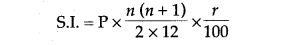where S.I. is the simple interest, P is the money deposited per month, n is the number of months for which the money has been deposited and r is the simple interest rate percent per annum.### Formulae Based Questions

Question 1. Naseem has a 5 years Recurring Deposit account in Punjab National Bank and deposit? Rs. 240 per month. If she receives Rs. 17,694 at the time of maturity find the rate of interest.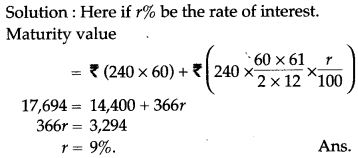Question 2. Zafarullah has a recurring deposit The list price of the television be? 12,500. account in a bank for 3½ years at 9.5% S.I. p.a. If he gets Rs. 78,638 at the time of maturity. Find the monthly instalment.Question 3. Mohan saves Rs. 25 per month from his pocket allowance and puts this saving every month in a bank recurring deposit scheme for a period of 72 months at 5.25%. What amount does he get on maturity?
Solution: See the table of Recurring deposit scheme. Here the month by instalment is Rs. 25 and the number of instalments is 72.
So the maturity value is the amount given in the table against the row marked 72 and the column marked 25. This amount is 2,721.90.
Hence, on maturity, Mohan gets Rs. 2,721.90.

Question 4. Using R.D., table calculate the values of a R.D., account of Rs. 80 for period of 9 months @ 11.5% p. a.
Solution: In the row of 80 we will locate the value under the column of 9 months which is 755.
So, maturity values of RD., account of 80 for 9 months @ 11.5% p.a Rs. 755.00.

Question 5. Veena deposits Rs. 100 per month in a bank cumulative time deposit scheme for a period of 5 years. What amount does she get on maturity if the rate of interest is 16%?
Solution: See the table of RD. scheme. For a monthly installment of Rs. 1oo per month the maturity values after 5 years is Rs. 8,447.80.

Question 6. Mrs. Goswami deposits Rs. 1000 every month in a recurring deposit account for 3 years at 8% interest per annum. Find the matured value.Question 7. Amit deposited Rs. 150 per month in a bank for 8 months under the Recurring Deposit Scheme. What will be the maturity value of his deposits, if the rate of interest is 8% per annum and interest is calculated at the end of every month?Question 8. Kiran deposited  200 per month for 36 months in a bank’s recurring deposit account. If the bank pays interest at the rate of 11% per annum, find the amount she gets on maturity.### Data Based Questions

Question 1. Given below are the entries in a Savings Bank A/c pass book:Calculate the interest for six months from February to July at 6% p.a.
Solution:Question 2. Mr. Dhoni has an account in the Union Bank of India. The following entries are from his pass book:Question 3. Anita opens an S.B. account in State Bank of India on August 1, 1983 with Rs. 100. She deposits Rs. 100 on the first or second day every month till and including February 1,1984. In between she withdrawal Rs. 200 on October 17,1983 and also on January 13,1984. Write the entries of the passbook.
Solution: The entries in the pass book are given below: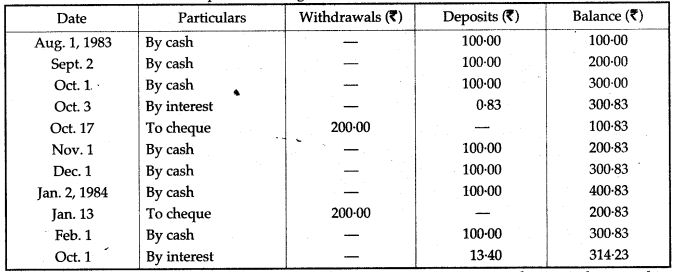Question 4. Akash, an employee of a bank, has a saving bank account in his bank that pays him
interest at the rate of 5% p.a., which is compounded every June and December. His pass book entries are as follow:Calculate the interest due at the end of June and find the balance on July 1, if he deposits a cash of ?100 on July 1, which is also entered immediately.
Solution: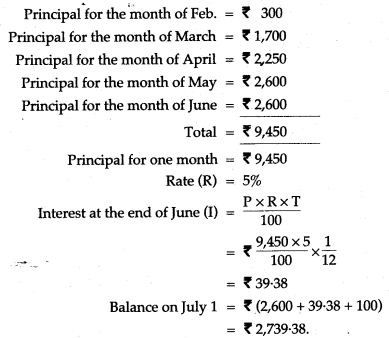Question 5. Mr. Chaudhary opened a Saving’s Bank Account at State Bank of India on 1st April 2007.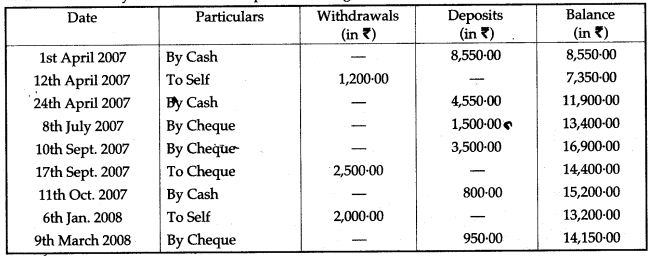If the bank pays interest at the rate of 5% per annum, find the interest paid on 1st April, 2008. Give your answer correct to the nearest rupee.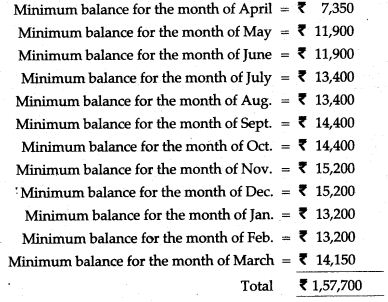Question 6. A page of Passbook of Mrs. C. Malik Savings Bank Account in year 2002 is given below:If the rate of interest decreases from 5% to 4% with effect from June 1st, 2002, compute the interest at the end of the year.
Solution: As per entries of the Passbook page of Mrs. C. Malik, we have: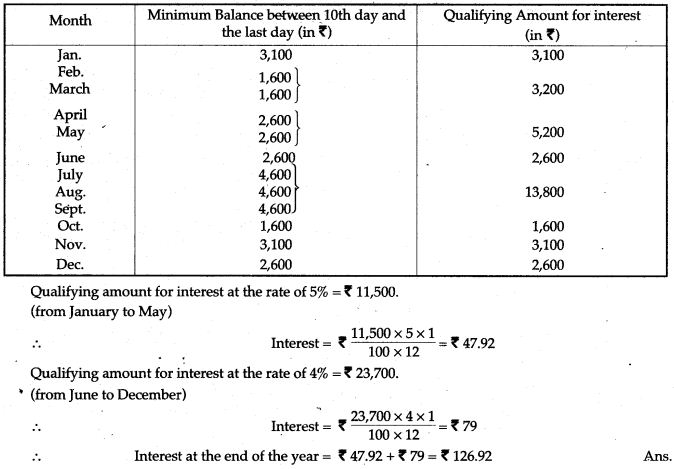Question 7. Suresh has joined a factory which pays wages by cheque only. He opens a S.B. account on Feb. 1, and his passbook has the following entries Upto 1st April of the year.He closes the account on 11th April. Complete the entries for 11th April at the rate of 5% p.a.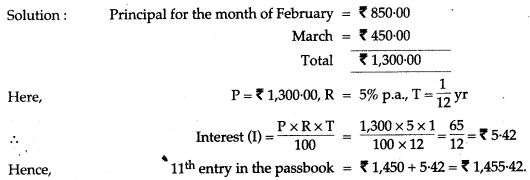Question 8. A page from the passbook of Mrs. Rama Bhalla is given below:
Calculate the interest due to Mrs. Bhalla for the period from January 2004 to December 2004, at the rate of 5% per annum.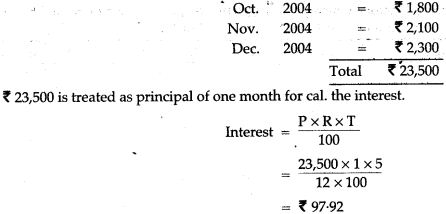Question 9. A page from the Savings Bank Account of Mr. Prateek is given below: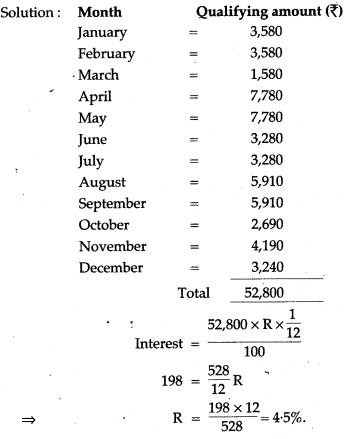Question 10. Mr. S.K. Mishra had a Savings Bank Account in Punjab National Bank. His Passbook had the following entries: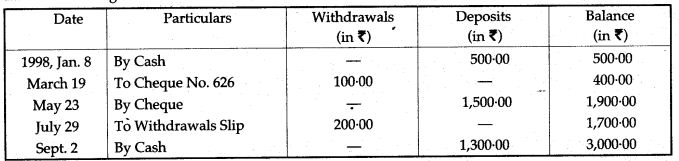If the interest is paid at the rate of 5% per annum at the end of September every year, calculate the amount he will get if he closes the account on October 30, of the same year.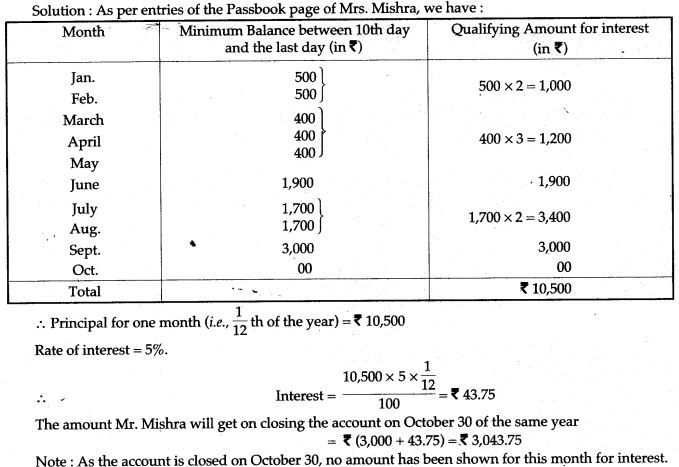Question 11. The entries in the passbook of a Saving Bank Account holder are as follows: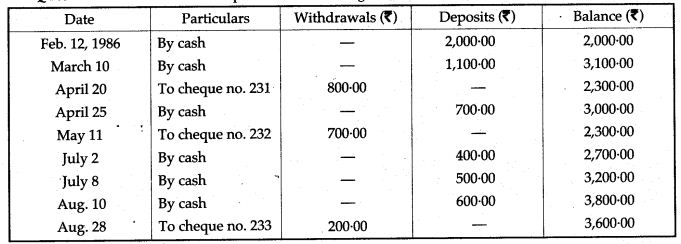(i) If the account is dosed on Sept 29, 1986, then month of Sept., will not earn interest and principal for one month Rs. 16,800.Question 12. Mrs. Kapoor opened a Savings Bank Account in State Bank of India on 9th January 2008. Her pass book entries for the year 2008 are given below: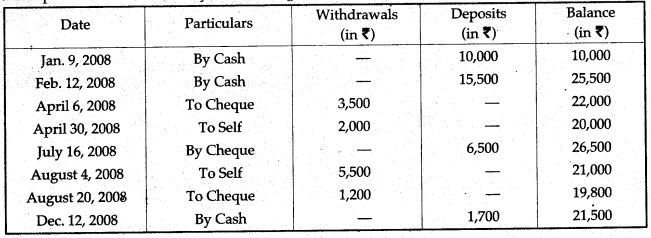Mrs. Kapoor closes the account on 31st December, 2008. If the bank pays interest at 4% per annum, find the interest Mrs. Kapoor receives on closing the account. Give your answer correct to the nearest rupee.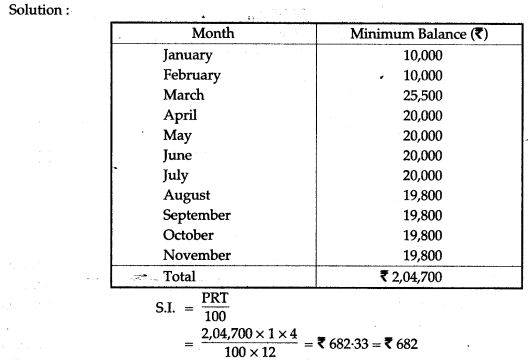Question 13. Mr. Ashok has an account in the Central Bank of India. The following entries are from his pass book: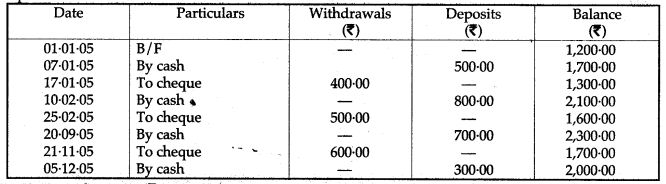If Mr. Ashok gets Rs. 83.75 as interest at the end of the year where the interest is compounded annually, calculate the rate of interest paid by the bank in his Savings Bank Account on 31st December, 2005.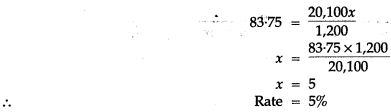Question 14. Mr. Mishra has a Savings Bank Account in Allahabad Bank. His pass book entries are as follows:Rate of interest paid by the bank is 4.5% per annum. Mr. Mishra closes his account on 30th October, 2007. Find the interest he receives.Question 15. A page from the saving bank account of Priyanka is given below:If the interest earned by Priyanka for the period ending September, 2006 is Rs. 175, find the rate of interest.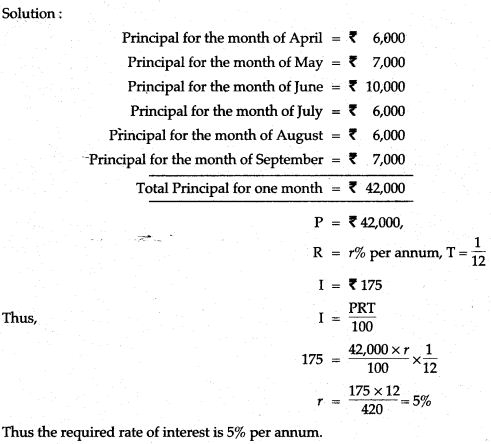Question 16. Given the following details, calculate the simple interest at the rate of 6% per annum up to June, 30: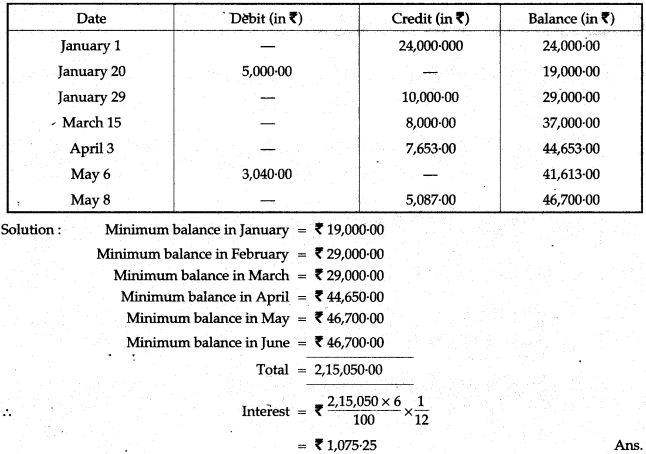For More Resources